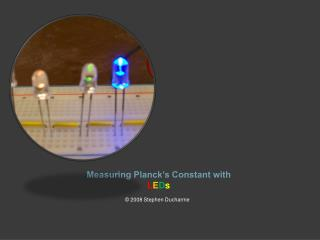DownloadDownload PresentationMeasuring Planck’s Constant with L E D s

Measuring Planck’s Constant with L E D s

Télécharger la présentationMeasuring Planck’s Constant with L E D s

- - - - - - - - - - - - - - - - - - - - - - - - - - - E N D - - - - - - - - - - - - - - - - - - - - - - - - - - -
Presentation Transcript

1. Measuring Planck’s Constant with LEDs © 2008 Stephen Ducharme

2. In 1900, German physicist Max Planck (1858-1947) was trying to model the broad smooth spectrum of electromagnetic radiation (i.e., light) emitted by a warm body. • This “black body radiation” is what you see coming from the sun, the filament of an incandescent light bulb, or a hot electric stove element. • Its ‘spectrum’, the range of frequencies making up the radiation, is readily displayed by a prism or a diffraction grating. • In explaining the shape of the black body spectrum, Planck assumed that the electromagnetic radiation came not in continuous waves of energy, but in discrete clumps of energy which we now call photons. • Planck postulated the ‘photons’, at each frequency have a discrete energy • E = hf, where E is the energy of the photon in Joules, f is the frequency in Hertz, and h is Planck’s constant.

3. Schematic and picture of LED circuit

4. STAR spectrophotometer. Scale Adjustment Slit Viewing Port

5. Adjusting your STAR spectrophotometer • Look through the viewing port with the slit pointing at a fluorescent ceiling light. • See If the green spectrum line is at 546 nm on the scale. • If needed, use scale adjustment to line up correctly. green spectrum line at 546 nm from the presence of Mercury vapor in the fluorescent bulb.

6. Measuring The LEDs wavelength • Adjust the current by turning the potentiometer so that the LEDs glow brightly. • look at each LED with the Spectrophotometer and measure the emission wavelength λ (in nanometers) of each. • Each person must record all data values on their own data sheet. • Please ask for help if you are having problems or are not sure you are doing things properly. For more information on LEDs go to the web site:

7. If the reading is not a definite line, read the middle and record this on your data chart. 620 nm

8. Voltage measurements. • Adjust the current by turning the potentiometer so that the LEDs are barely visible, thus ensuring that the LEDs are operating near their threshold voltage(the minimum voltage necessary to generate photons). • Measure the threshold voltage V (in Volts) across each LED. • Record each voltage on your data sheet.

9. Calculating Planck’s constant Here is an example using the UV LED as an example. • First we will find the Energy (E) , and then the frequency (f) • E = eV • E = (1.60 X 10-19 C)(2.876 V) • E = 4.60 X 10-19 J • f = c/λ , what should “c” have for units to get Hertz in this problem? • f = (3.00 X 1017 nm/s) / (390 nm) • f = 7.69 X1014 Hz

10. Now, we will calculate Planck’s constant (h) for the UV LED. • h = E / f • h = 4.60 X 10-19 J / 7.69 X 1014 Hz • h = 5.98 X 10-34 J/Hz • What is the actual value for Planck’s constant? • How close was this calculation to that number?

11. This activity you will need the following Equipment: Table Notes: (1) IR = Infrared, R = red, Y = yellow, O = Orange, G = Green, B = Blue. Other colors may be available. (2)Digikey(800) 344-4539. Edmund Scientific (609) 573-6250. Pasco (800) 772-8700. STAR Spectrometer, Model PS-14 plastic from Learning Technologies Project (800) 537-8703, or model OA-160 from the Astronomical Society of the Pacific (800) 335-2624 or http://www.aspsky.org/aspcat/observ2.html#proj. Mouser Electronics (800) 346-6873. You can find similar items from other vendors, including standard educational physics equipment suppliers Pasco, KEP, Jameco, and others. Radio Shack has all the necessary Electronic components and a selection of multimeters as do Digikey, Mouser, and Newark Electronics. Call for Catalogs today!

12. Other lessons that could be taught or reviewed with this experiment: • Simple Schematics • Series and Parallel Circuits • Measuring Total Voltage in a Series Circuit. • VTotal= V1 + V2 + V3 ….. • Measuring Resistance of the LEDs using Ohm’s Law. • R = V/ I • Variable Resistors • Diodes • Batteries in Series